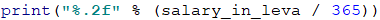# Chapter 2.2. Simple Calculations – Exam Problems

In the previous chapter, we explained how to work with the system console – how to read numbers from it and how to print the output. We went through the main arithmetical operations and briefly mentioned data types. Now, we are going to practice what we have learned by solving a few more complex exam problems.

## Reading Numbers from The Console

Before going to the tasks, we are going to revise the most important aspects of what we have studied in the previous chapter. We will start by reading numbers from the console.

We need to create a variable to store the integer (for example, num) and use a standard command for reading input from the console (the function input(…)), combined with the function int(…) which converts a string to an integer:

num = int(input())


We read a floating-point number, the same way we read an integer one, but this time we use the function float(…):

num = float(input())


## Printing Text Using Placeholders

A placeholder is an expression that is replaced with a particular value while printing an output. The function print(…) supports printing a string based on a placeholder, there are a few ways to do that, as we reviewed in the previous chapter:

• The first argument that we pass to the function is the formatted string, followed by the symbol % and the number of arguments, equal to the number of placeholders, placed in brackets:
print("You are %s %s, a %d-years old person from %s." % ("Ivan", "Ivanov", "16", "Burgas"))

• The second way is the so-called "f-strings", where before the formatted string we put the symbol f and the arguments are in the string between curled brackets {}:
first_name = "Ivan"
last_name = "Ivanov"
age = 21
town = "Burgas"
print(f"You are {first_name} {last_name}, a {age}-years old person from {town}.")


There are other ways to format a string and the more curious of you can check them in the following article, as well as find the difference between them:https://cito.github.io/blog/f-strings/

## Arithmetic Operators

Let's revise the main arithmetic operators for simple calculations.

### Operator +

result = 3 + 5 # the result is 8


### Operator -

result = 3 - 5 # the result is -2


### Operator *

result = 3 * 5 # the result is 15


### Operator \/ and \/\/

result = 5 / 2 # the result is 2.5 (fractional division)
result2 = 7 // 3 # the result is 2 (integer division)


## Concatenation

By using the operator + between string variables (or between a string and a number), concatenation is being performed (combining strings):

firstName = "Ivan";
lastName = "Ivanov";
age = 19;
str = firstName + " " + lastName + " is " + age + " years old";
# Ivan Ivanov is 19 years old


## Exam Problems

Now, after having revised how to make simple calculations and how to read and print numbers from the console, let's go to the tasks. We will solve a few problems from a SoftUni practical exam.

## Problem: Training Lab

A training lab has a rectangular size l x w meters, without columns on the inside. The hall is divided into two parts- left and right, with a hallway approximately in the middle. In both parts, there are rows with desks. In the back of the hall, there is a big entrance door. In the front, there is a podium for the lecturer. A single working place takes up 70 x 120 cm (a table with size 70 x 40 cm + space for a chair and passing through with size 70 x 80 cm). The hallway width is at least 100 cm. It is calculated that due to the entrance door (which has 160 cm opening) exactly one working space is lost, and due to the podium (which has a size of 160 x 120 cm) exactly two working spaces are lost. Write a program that reads the size of the training lab as input parameters and calculates the number of working places in it (look at the figure).

### Input Data

Two numbers are read from the console, one per line: l (length in meters) and w (width in meters).

Constraints: 3 ≤ w ≤ l ≤ 100.

### Output Data

Print an integer in the console: the number of working places in the training lab.

### Sample Input and Output

Input Output Figure
15
8.9
129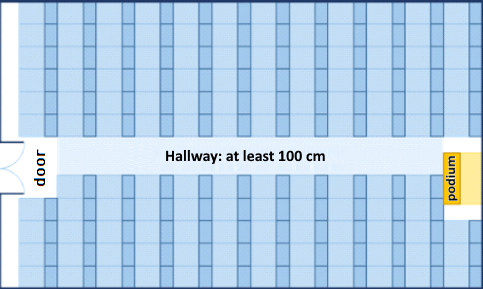8.4
5.2
39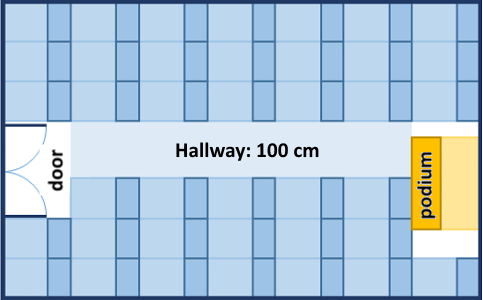#### Clarification of The Examples

In the first example, the hall length is 1500 cm. 12 rows can be situated in it (12 120 cm = 1440 + 60 cm difference). The hall width is 890 cm. 100 cm of them are for the hallway in the middle. The rest 790 cm can be situated by 11 desks per row (11 \ 70 cm = 770 cm + 20 cm difference). Number of places = 12 * 11 - 3 = 132 - 3 = 129 (we have 12 rows with 11 working places = 132 minus 3 places for podium and entrance door).

In the second example, the hall length is 840 cm. 7 rows can be situated in it (7 * 120 cm = 840, no difference). The hall width is 520 cm. 100 cm from them is for the hallway in the middle. The rest 420 cm can be situated by 6 desks per row (6 * 70 cm = 420 cm, no difference). Number of places = 7 * 6 - 3 = 42 - 3 = 39 (we have 7 rows with 6 working places = 42 minus 3 places for podium and entrance door).

### Hints and Guidelines

Try to solve the problem on your own first. If you do not succeed, go through the hints.

#### Idea for Solution

As with any programming task, it is important to build an idea for its solution, before having started to write code. Let's carefully go through the problem requirements. We have to write a program that calculates the number of working places in a training lab, where the number depends on the hall length and height. We notice that the provided input data will be in meters and the information about how much space the working places and hallway take, will be in centimeters. To do the calculations, we will use the same measuring units, no matter whether we choose to convert length and height into centimeters or the other data in meters. The first option is used for the presented solution.

Next, we have to calculate how many columns and how many rows with desks will fit. We can calculate the columns by subtracting the width by the necessary space for the hallway (100 cm) and dividing the difference by 70 cm (the length of a workplace). We find the rows by dividing the length by 120 cm. Both operations can result in a real number with a whole and a fractional part, but we have to store only the whole part in a variable. In the end, we multiply the number of rows by the number of columns and divide it by 3 (the lost places for entrance door and podium). This is how we calculate the needed value.

#### Choosing Data Types

From the example, we see that a real number with whole and fractional parts can be given as an input, therefore, it is not appropriate to choose data type int. This is why we use float. Choosing a data type for the next variables depends on the method we choose to solve the problem. As with any programming task, this one has more than one way to be solved.

#### Solution

It is time to go to the solution. We can divide it into three smaller tasks:

• Reading input from the console.
• Doing the calculations.
• Printing the output on the console.

The first thing we have to do is read the input from the console. With input(…) we read the values from the console and with the function float(…) string is converted into float.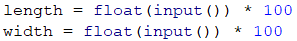Let's move to the calculations. The special part here is that after having divided the numbers, we have to store only the whole part of the result in a variable.Search in Google! Whenever we have an idea how to solve a particular problem, but we do not know how to write it in Python or we are dealing with one that many other people have had before us, the easiest way to solve it is by looking for information on the Internet.

In this case, we can try with the following search: "Python gets whole number part of float". One possible way is to use the method math.trunc(…) and don't forget to refer to the math library. The code down below is blurred on purpose and it should be completed by the reader:With print(…) we print the result in the console: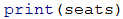## Problem: Vegetable Market

A gardener is selling his harvest on the vegetable market. He is selling vegetables for N BGN per kilogram and fruits for M BGN per kilogram. Write a program that calculates the earnings of the harvest in Euro. (Assume that 1 EUR is equal to 1.94 BGN).

### Input Data

Four numbers are read from the console, one per line:

• First line: Vegetable price per kilogram – a floating-point number.
• Second line: Fruit price per kilogram – a floating-point number.
• Third line: Total kilograms of vegetables – an integer.
• Fourth line: Total kilograms of fruits – an integer.

Constraints: all numbers will be within the range from 0.00 to 1000.00.

### Output Data

Print on the console one floating-point number: the earnings of all fruits and vegetables in EUR.

### Sample Input and Output

Input Output
0.194
19.4
10
10
101

Clarification for the first example:

• Vegetables cost: 0.194 BGN. * 10 kg. = 1.94 BGN.
• Fruits cost: 19.4 BGN. * 10 kg. = 194 BGN.
• Total: 195.94 BGN. = 101 EUR.
Input Output
1.5
2.5
10
10
20.6185567010309

### Hints and Guidelines

First, we will give a few ideas, followed by particular hints for solving the problem and the essential part of the code.

#### Idea for Solution

Let's first go through the problem requirements. In this case, we have to calculate the total income from the harvest. It equals the sum of the earnings from the fruits and vegetables which we can calculate by multiplying the price per kilogram by the quantity. The input is given in BGN and the output should be in euros. It is assumed that 1 EUR equals 1.94 BGN, therefore, to get the wanted output value, we have to divide the sum by 1.94.

#### Choosing Data Types

After we have a clear idea of how to solve the task, we can continue with choosing appropriate data types. Let's go through the input: we have two integers for total kilograms of vegetables and fruits, therefore, the variables we declare to store their values will be of int type. The prices of the fruits and vegetables are said to be two floating-point numbers and therefore, the variables will be of float type.

We can also declare two variables to store the income from the fruits and vegetables separately. The output should be a floating-point number, so the result should be float type.

#### Solution

It is time to get to the solution. We can divide it into three smaller tasks:

• Reading input from the console.
• Doing the calculations.
• Printing the output on the console.

To read the input, we declare variables, which we have to name carefully so that they can give us a hint about the values they store. With input(…), we read values from the console and with the functions int(…) and float(…), we convert the particular string value into int and double: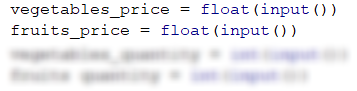We do the necessary calculations: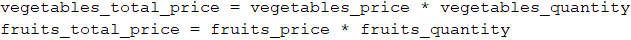The task does not specify a special output format, therefore, we just have to calculate the requested value and print it on the console. As in mathematics and so in programming, the division has a priority over addition. However, in this task, first, we have to calculate the sum of the two input values and then divide by 1.94. To give priority to addition, we can use brackets. With print(…) we print the output on the console: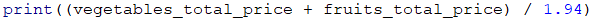## Problem: Change Tiles

On the ground in front of an apartment building tiles need to be placed. The ground has a square shape with a side of N meters. The tiles are "W" meters wide and "L" meters long. There is one bench on the ground with a width of "M" meters and a length of "O" meters. There is no need to place tiles under it. Each tile is replaced for 0.2 minutes.

Write a program that reads from the console the size of the ground, the tiles and the bench, and calculates how many tiles are needed to cover the ground and what is the total time for placing all of the tiles.

Example: ground with size 20 m has an area of 400 $m^2$. A bench that is 1 m wide and 2 m long, has an area of 2 $m^2$. One tile is 5 m wide and 4 m long and has an area of 20 $m^2$. The space that needs to be covered is 400 - 2 = 398 $m^2$. 398 / 20 = 19.90 tiles are necessary. The time needed is 19.90 * 0.2 = 3.98 minutes.

### Input Data

The input data comes as 5 numbers, which are read from the console:

• N – length of a side of the ground within the range of [1 … 100].
• W – width per tile within the range of [0.1 … 10.00].
• L – length per tile within the range of [0.1 … 10.00].
• M – width of the bench within the range of [0 … 10].
• O – length of the bench within the range of [0 … 10].

### Output Data

Print on the console two numbers:

• number of tiles needed for the repair
• total time for placing them

Each number should be on a new line and rounded to the second decimal place.

### Sample Input and Output

Input Output
20
5
4
1
2
19.9
3.98

Explanation of the example:

• Total area = 20 * 20 = 400.
• Area of the bench = 1 * 2 = 2.
• Area for covering = 400 – 2 = 398.
• Area of tiles = 5 * 4 = 20.
• Needed tiles = 398 \/ 20 = 19.9.
• Needed time = 19.9 * 0.2 = 3.98.
Input Output
40
0.8
0.6
3
5
3302.08333333333
660.416666666667

### Hints and Guidelines

Let's make a draft to clarify the task requirements. It can look the following way: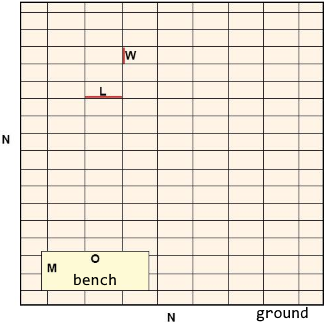#### Idea for Solution

It is required to calculate the number of tiles that have to be placed, as well as the time for placing them. To find that number, we have to calculate the area that needs to be covered and divide it by the area per tile. By the requirements of the problem, the ground is square, therefore, we find the total area by multiplying its side by its value N * N. After that, we calculate the area that the bench takes up by multiplying its two sides as well M * O. After subtracting the area of the bench from the area of the whole ground, we obtain the area that needs to be repaired.

We calculate the area of a single tile by multiplying its two sides with one another W * L. As we already stated, now we have to divide the area for covering by the area of a single tile. This way, we find the number of necessary tiles. We multiply it by 0.2 (the time needed for changing a tile). Now, we have the wanted output.

#### Choosing Data Types

The length of the side of the ground, the width and the length of the bench will be given as integers, therefore, to store their values, we can use the system function int(…). We will be given floating-point numbers for the width and the length of the tiles and this is why we will use float(…).

#### Solution

As in the previous tasks, we can divide the solution into three smaller tasks:

• Doing the calculations.
• Printing the output on the console.

The first thing we have to do is go through the input of the task. It is important to pay attention to the sequence they are given in. With input(…) we read values from the console and with int(…) and float(…), we convert the particular string value into int or float: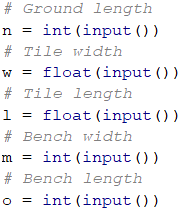After we have initialized the variables and have stored the corresponding values in them, we move to the calculations. The code below is blurred on purpose, so the reader can think by himself over it: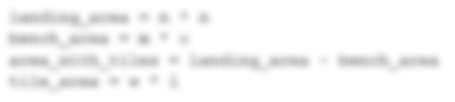We calculate the values that we have to print on the console. The number of necessary tiles is obtained by dividing the area that needs to be covered by the area of a tile: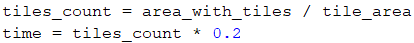In the task is specified that the number of the output should be rounded to the second decimal place. That is why we cannot just print the value with print(…). We will use the method round(…), which is rounding to the closest integer number with exactly n-numbers after the decimal place. For example, the number 1.35 will be rounded to 1, and 1.65 - to 2: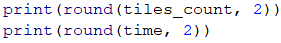## Problem: Money

Some time ago, Pesho bought Bitcoins. Now, he is going on vacation in Europe and he needs euros. Apart from Bitcoins, he has Chinese yuans as well. Pesho wants to exchange his money for euros for the tour. Write a program that calculates how much euro he can buy, depending on the following exchange rates:

• 1 Bitcoin = 1168 BGN.
• 1 Chinese yuan (CNY) = 0.15 USD.
• 1 USD = 1.76 BGN.
• 1 EUR = 1.95 BGN.

The exchange office has a commission fee of 0% to 5% from the final sum in euro.

### Input Data

Three numbers are read from the console:

• On the first line – number of Bitcoins. Integer within the range of [0 … 20].
• On the second line – number of Chinese yuans. Floating point number within the range of [0.00 … 50 000.00].
• On the third line – commission fee. Floating point number within the range of [0.00 … 5.00].

### Output Data

Print one number on the console – the result of the exchange of currencies. The output should be rounded to the second decimal place.

### Sample Input and Output

Input Output
1
5
5
569.67

Explanation:

• 1 Bitcoin = 1168 BGN
• 5 Chinese yuan (CNY) = 0.75 USD
• 0.75 USD = 1.32 BGN
• 1168 + 1.32 = 1169.32 BGN = 599.651282051282 EUR
• Commission fee: 5% of 599.651282051282 = 29.9825641025641
• Result: 599.651282051282 - 29.9825641025641 = 569.668717948718 = 569.67 EUR
Input Output Input Output
20
5678
2.4
12442.24 7
50200.12
3
10659.47

### Hints and Guidelines

Let's first think of the way we can solve the task, before having started to write code.

#### Idea for Solution

We see that the number of bitcoins and the number of Chinese yuans will be given in the input. The output should be in euros. The exchange rates that we have to work with are specified in the task. We notice that we can only exchange the sum in BGN to EUR, therefore, we first have to calculate the whole sum that Pesho has in BGN, and then calculate the output.

As we have information for the exchange rate of Bitcoins to BGN, we can directly exchange them. On the other hand, to get the value of Chinese yuans in BGN, first, we have to exchange them in USD, and then the USD to BGN. Finally, we will sum the two values and calculate how much euro that is.

Only the final step is left: calculating the commission fee and subtracting the new sum from the total one. We will obtain a floating-point number for the commission fee, which will be a particular percent of the total sum. Let's divide it by 100, to calculate its percentage value. Then we will multiply it by the sum in euro and divide the result from the same sum. Print the final sum on the console.

#### Choosing Data Types

Bitcoins are given as an integer, therefore, we can declare a variable using the int(…) function. For Chinese yuan and commission fee, we obtain a floating-point number, therefore, we are going to use float(…).

#### Solution

After we have built an idea on how to solve the task and we have chosen the data structures that we are going to use, it is time to get to writing the code. As in the previous tasks, we can divide the solution into three smaller tasks:

• Reading input from the console.
• Doing the calculations.
• Printing the output on the console.

We declare the variables that we are going to use and again we have to choose meaningful names, which are going to give us hints about the values they store. We initialize their values: we create variables, where we will store the string arguments passed to the function and convert them to int or double: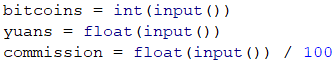Then we do the necessary calculations: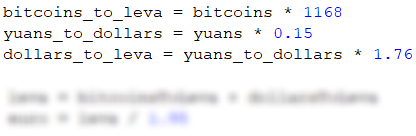Finally, we calculate the commission fee and subtract it from the sum in euro. Let's pay attention to the way we could write this: euro -= commission * euro is the short way to write euro = euro - (commission * euro). In this case, we use a combined assignment operator (-=) that subtracts the value of the operand to the right from the one to the left. The operator for multiplication (*) has a higher priority than the combined operator, this is why the expression commission * euro is performed first and then its value is divided.

Finally, we have to print the result in the console. We should notice that we have to format the output to the second decimal place. The difference between this and the previous task is that here, even if the number is an integer, we have to print it to the second decimal place (for example 5.00). One way to do so is to use the function print(…) and to format a string using a placeholder(%.2f). By using it, we can covert a number into a string, saving the specified numbers after the decimal point: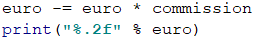Let's pay attention to something that applies to all other problems of this type: written like that, the solution of the task is pretty detailed. As the task itself is not too complex, in theory, we could write one big expression, where right after having taken the input, we calculate the output. For example, such an expression would look like this: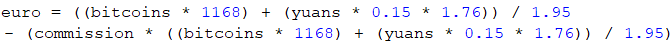This code would print a correct result, but it is hard to read. It won't be easy to find out how it works and whether it contains any mistakes, as well as finding such and correcting them. Instead of one complex expression, it is better to write a few simpler ones and store their values in variables with appropriate names. This way, the code is cleaner and easily maintainable.

## Problem: Daily Earnings

Ivan is a programmer in an American company, and he works at home approximately N days per month by earning approximately M USD per day. At the end of the year, Ivan gets a bonus, which equals 2.5 of his monthly salary. In addition, 25% of his annual salary goes for taxes. Write a program that calculates what is the amount of Ivan's net average earnings in BGN per day, as he spends them in Bulgaria. It is accepted that one year has exactly 365 days. The exchange rate of US USD to BGN will be passed to a function.

### Input Data

Three numbers are read from the console.

• On the first line – workdays per month. An integer within the range of [5 … 30].
• On the second line – daily earnings. A floating-point number within the range of [10.00 … 2000.00].
• On the third line – exchange rate of USD to BGN /1 dollar = X BGN/. A floating-point number within the range of [0.99 … 1.99].

### Output Data

Print one number on the console – the average daily earnings in BGN. The result will be rounded up to the second digit after the decimal point.

### Sample Input and Output

Input Output
21
75.00
1.59
74.61

Explanation:

• One monthly salary = 21 * 75 = 1575 USD.
• Annual income = 1575 * 12 + 1575 * 2.5 = 22837.5 USD.
• Taxes = 25% of 22837.5 = 5709.375 USD.
• Net annual income in USD = 17128.125 USD = 27233.71875 BGN.
• Average earnings per day = 27233.71875 / 365 = 74.61 BGN.
Input Output Input Output
15
105
1.71
80.24 22
199.99
1.50
196.63

### Hints and Guidelines

Firstly, we have to analyze the task and think of a way to solve it. Then, we will choose data types and, finally, we will write the code.

#### Idea for Solution

Let's first calculate how much the monthly salary of Ivan is. We do that by multiplying the working days per month by his daily earnings. We multiply the result by 12, to calculate his salary for 12 months, and then, we multiply it by 2.5, so that we can calculate the bonus. After having summed up the two values, we calculate his annual income. Then, we will reduce the annual income by 25% taxes. We can do that by multiplying the total income by 0.25 and subtracting the result out of it. Depending on the exchange rate, we exchange the USD to BGN and after that, we divide the result by 365.

#### Choosing Data Types

The working days per month are given as an integer, therefore, we can convert the string into an int with the function int(…). For both the earned money and the exchange rate of USD to EUR, we will obtain a floating-point number, therefore, we will use the function float(…).

#### Solution

Again: after we have an idea of how to solve the problem and we have considered the data types that we are going to use, we can start writing the program. As in the previous tasks, we can divide the solution into three smaller tasks:

• Doing the calculations.
• Printing the output on the console.

Then we declare the variables that we are going to use by trying to choose meaningful names. We create a variable to store the arguments passed to the function, by converting the string to integer or floating number by using int(…)/float(…):After that we do the calculations: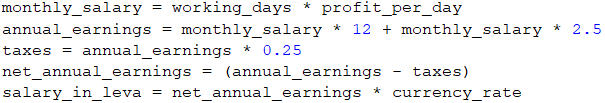We could write an expression that calculates the annual income without brackets as well. As multiplication is an operation that has a higher priority over addition, it will be performed first. Despite that, writing brackets is recommended when using more operators, as this way the code is easily readable and chances of making a mistake are smaller.

Finally, we have to print the result on the console. We notice that the number has to be rounded up to the second digit after the decimal point. To do that, we can use the same placeholder, just like the previous task: